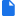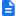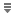# Code Examples

• An example of using our R to solve a jelly bean problem.jellybeans.R

• What do Boulder residents complain about?simple_table_operations.R

• Code:SexBiasUCB.R

• Data:UCBAdmissions.csv

• Random Sampling:
• Code:random_sample.R

• Data:R11452947_SL140.csv

• Normal distributions from random samples
• Coin Flipping: Random Chance and Bias
•Flipping.R

• Simple Box Models: Rainfall in Boulder
•Rainfall_Estimates.R

• Margin of Error
• Tests of significance: Home-brewed Z-Test
•Making_a_Z-Test.R

•Exploring Two Sample Tests.R

•Simple Two Sample Test.R

• Correlation (by hand)
• Regression Examples
• Simple regression and Derivation of R-Square
•Regresion_demo.R

• Data
•GA_Votes.csv

• Uncertainty in Model Parameters
• Regression with Spatial Data
•Regression_With_Spatial_Data.R

•ElectionData_04.zip